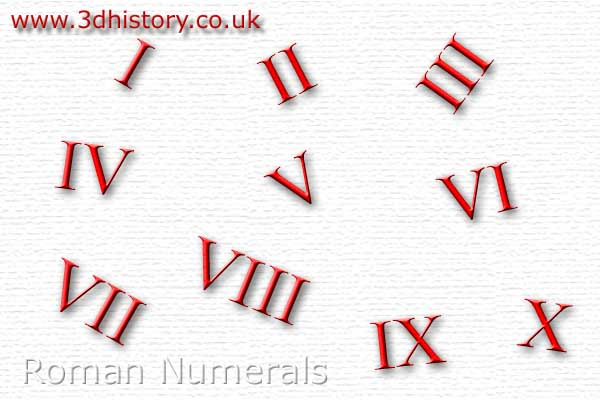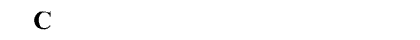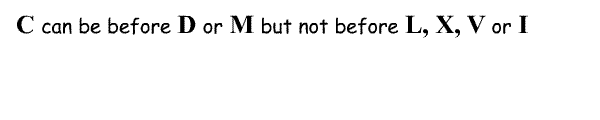# Roman NumeralsThe Romans used a different system of numbers to the one we use today; instead of the digits 0 to 9 they used symbols to represent different values: Can you convert Roman to Modern numbers? Try the Quiz
 Roman Numerals I = 1 V = 5 X = 10 L = 50 C = 100 D = 500 M = 1000
 The symbols are normally arranged in descending order and the values are simply added together.Where a symbol of lesser value comes before a symbol of greater value then the lesser value is subtracted. For example IV actually means 5 - 1 = 4 and CMII means 1000 - 100 + 1 + 1 = 902 Only C, X and I can be subtracted and only one smaller symbol can be used. The symbol used for subtraction must not be less than a tenth of the value of the number it precedes. So something like IIX or LM is NOT allowed. (IIX uses two smaller numbers (II) before the larger one (X) and L(50) is less than one tenth the size of M(1000))
 This means that:Try this Quiz to see if you work out the numbers